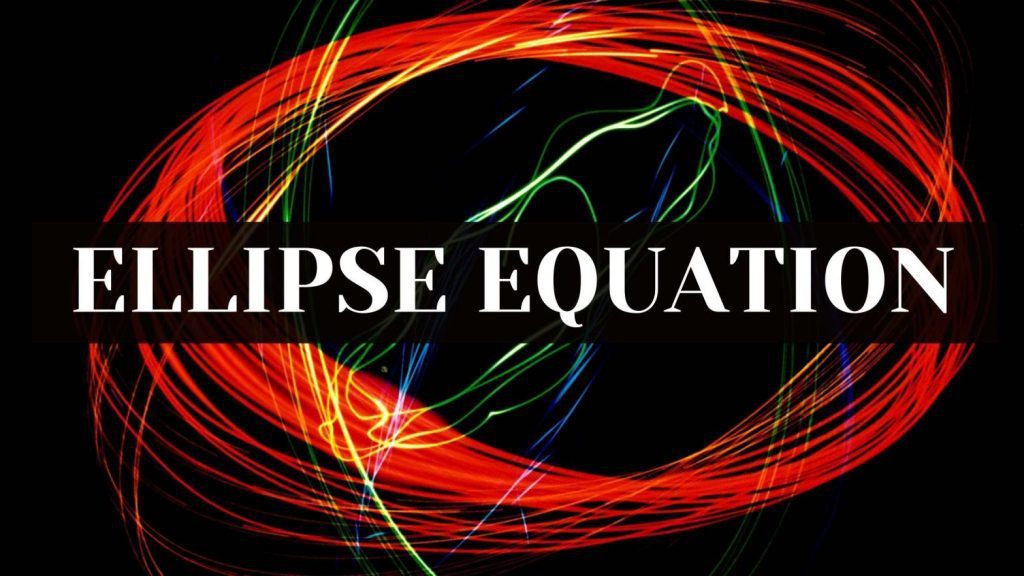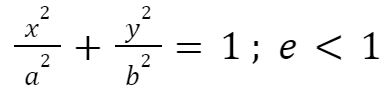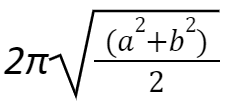Maths
Study Material

# Ellipse – Equation

An ellipse represents the locus of the points, the sum of whose distances from the two foci (the two fixed points) of the ellipse is a constant value.

6 minutes long
Posted by Kunduz Tutor, 7/11/2021Hesap Oluştur

Got stuck on homework? Get your step-by-step solutions from real tutors in minutes! 24/7. Unlimited.

Ellipse is a crucial topic of the conic section which comprises an integral part of it. Ellipse is oval in shape, otherwise most of its properties are similar to a circle. We can understand ellipse by considering some simple real-life examples of the ellipse in our daily-to-day life; the shape of an egg in a two-dimensional (2-D) form, the track field of athletics in a sports stadium, the orbits of satellites and planets. Here we will learn more about Ellipse and Ellipse equation.

Author – Ojasvi Chaplot

## What is an Ellipse?

An ellipse represents the locus of the points, the sum of whose distances from the two foci (the two fixed points) of the ellipse is a constant value.

Or

In some sense, an ellipse is the set of all the points in a plane, the sum of whose distances from the two fixed points, which are called as the foci (plural of focus), in the plane is constant.

## Basic Terms of an Ellipse

• Foci of Ellipse The coordinates of the two foci an ellipse are F(c,0) and F'(-c,0). And hence, the distance between them is 2c.
• Center of Ellipse – The mid-point of the line segment joining the foci is called the center of the ellipse
• Major Axis of Ellipse – The line segment through the foci of the ellipse is called the major axis.
• We denote the length of the major axis by 2a
• Minor Axis of Ellipse – The line segment through the center and perpendicular to the major axis is called the minor axis.
• We denote the length of the minor axis by 2b
• Vertices of Ellipse –  The end points of the major axis are called the vertices of the ellipse.
• Eccentricity of an Ellipse – The eccentricity of an ellipse is the ratio of the distances from the center of the ellipse to one of the foci and to one of the vertices of the ellipse. The eccentricity is also known as the degree of flatness.
• The eccentricity of an ellipse is less than one (i.e. e < 1).
• Represented as eccentricity, e=c/a
• Latus Rectum of Ellipse – The latus rectum of the ellipse is a line segment perpendicular to the major axis through any of the foci and whose end points lie on the ellipse.
• The length of the latus rectum of the ellipse is 2b2/a.

## Ellipse Equation

The general equation of the ellipse can be used to represent an ellipse algebraically in the coordinate plane. The ellipse equation can be written as,### Area of Ellipse

Area of the ellipse = π × semi-major axis × semi-minor axis

Hence, Area of the ellipse = πab

### Perimeter of Ellipse

Perimeter of the ellipse is given as :## Standard Ellipse Equation(s)

The standard ellipse equation(s) and their various properties and formulas related to the ellipse equation are explained through the table given below–

## Derivation of Ellipse Equation

The derivation of the ellipse equation can be shown as follows:

For deriving the ellipse equation, first of all what we do is, we derive the relationship between the semi-major axis (a), semi-minor axis (b) and the distance of the focus from the center (c). The primary aim is to find out the relationship between a, b and c.

As we know that,

• The length of the major axis of the ellipse = 2a
• The length of the minor axis of the ellipse = 2b. The distance between the foci = 2c.

Also , Let us take a point P at one end of the major axis and aim to find out the sum of the distances of the point P from each of the foci F and F’.

• PF + PF’ = OP – OF + OF’ + OP = a – c + c + a
• PF + PF’ = 2a

Now, let’s take another point Q at one end of the minor axis and aim to find out the sum of the distances of the point Q from each of the foci F and F’.

• QF + QF’ = √(b2 + c2) + √(b2 + c2)
• QF + QF’ = 2√(b2 + c2)

Since, the points P and Q lie on the ellipse and also, the definition of the ellipse says that for any point on the ellipse, the sum of the distances from the two foci (the two fixed points) is a constant value.

• 2√(b2 + c2) = 2a => √(b2 + c2) = a
• b2 + c2 = a2 => c2 = a2 – b2

Let us consider a point S(x, y) on the ellipse such that,

SF + SF’ = 2a

##### Now, by using the distance formula

we have,

• √{(x + c)2 + y2} + √{(x – c)2 + y2} = 2a
• √{(x + c)2 + y2} = 2a – √{(x – c)2 + y2}

Further, on squaring both the sides, we get

• (x + c)2 + y2 = 4a2 – 4a√{(x – c)2 + y2} + (x – c)2 + y2

On simplifying the above equation, we get

• √{(x – c)2 + y2} = a – x(c/a)

Again, on squaring both the sides and simplifying it further, we get

• x2/a2 + y2/(a2 – c2) = 1

As we know that, c2 = a2 – b2

Therefore, on substituting it, we get the result as

• x2/a2 + y2/b2 = 1

Thus, this derives the standard ellipse equation: x2/a2 + y2/b2 = 1

## Special Cases of Ellipse Equation

• If c = 0, then both the foci get merged together with the center of the ellipse. And, also a2 becomes equal to b2, i.e., a = b. Hence, in this case, the ellipse becomes a circle.
• If c = a, then b becomes 0. Therefore, a line segment joining both the foci is obtained.

## Picked For You

Furthermore, these are some topics which might interest you: